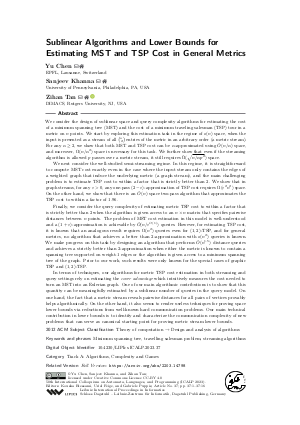Document# Sublinear Algorithms and Lower Bounds for Estimating MST and TSP Cost in General Metrics

### Authors Yu Chen, Sanjeev Khanna, Zihan Tan## File

LIPIcs.ICALP.2023.37.pdf
• Filesize: 0.75 MB
• 16 pages

## Cite As

Yu Chen, Sanjeev Khanna, and Zihan Tan. Sublinear Algorithms and Lower Bounds for Estimating MST and TSP Cost in General Metrics. In 50th International Colloquium on Automata, Languages, and Programming (ICALP 2023). Leibniz International Proceedings in Informatics (LIPIcs), Volume 261, pp. 37:1-37:16, Schloss Dagstuhl - Leibniz-Zentrum für Informatik (2023)
https://doi.org/10.4230/LIPIcs.ICALP.2023.37

## Abstract

We consider the design of sublinear space and query complexity algorithms for estimating the cost of a minimum spanning tree (MST) and the cost of a minimum traveling salesman (TSP) tour in a metric on n points. We start by exploring this estimation task in the regime of o(n) space, when the input is presented as a stream of all binom(n,2) entries of the metric in an arbitrary order (a metric stream). For any α ≥ 2, we show that both MST and TSP cost can be α-approximated using Õ(n/α) space, and moreover, Ω(n/α²) space is necessary for this task. We further show that even if the streaming algorithm is allowed p passes over a metric stream, it still requires Ω̃(√{n/α p²}) space. We next consider the well-studied semi-streaming regime. In this regime, it is straightforward to compute MST cost exactly even in the case where the input stream only contains the edges of a weighted graph that induce the underlying metric (a graph stream), and the main challenging problem is to estimate TSP cost to within a factor that is strictly better than 2. We show that in graph streams, for any ε > 0, any one-pass (2-ε)-approximation of TSP cost requires Ω(ε² n²) space. On the other hand, we show that there is an Õ(n) space two-pass algorithm that approximates the TSP cost to within a factor of 1.96. Finally, we consider the query complexity of estimating metric TSP cost to within a factor that is strictly better than 2 when the algorithm is given access to an n × n matrix that specifies pairwise distances between n points. The problem of MST cost estimation in this model is well-understood and a (1+ε)-approximation is achievable by Õ(n/ε^{O(1)}) queries. However, for estimating TSP cost, it is known that an analogous result requires Ω(n²) queries even for (1,2)-TSP, and for general metrics, no algorithm that achieves a better than 2-approximation with o(n²) queries is known. We make progress on this task by designing an algorithm that performs Õ(n^{1.5}) distance queries and achieves a strictly better than 2-approximation when either the metric is known to contain a spanning tree supported on weight-1 edges or the algorithm is given access to a minimum spanning tree of the graph. Prior to our work, such results were only known for the special cases of graphic TSP and (1,2)-TSP. In terms of techniques, our algorithms for metric TSP cost estimation in both streaming and query settings rely on estimating the cover advantage which intuitively measures the cost needed to turn an MST into an Eulerian graph. One of our main algorithmic contributions is to show that this quantity can be meaningfully estimated by a sublinear number of queries in the query model. On one hand, the fact that a metric stream reveals pairwise distances for all pairs of vertices provably helps algorithmically. On the other hand, it also seems to render useless techniques for proving space lower bounds via reductions from well-known hard communication problems. Our main technical contribution in lower bounds is to identify and characterize the communication complexity of new problems that can serve as canonical starting point for proving metric stream lower bounds.

## Subject Classification

##### ACM Subject Classification
• Theory of computation → Design and analysis of algorithms
##### Keywords
• Minimum spanning tree
• travelling salesman problem
• streaming algorithms

## Metrics

• Access Statistics
• Total Accesses (updated on a weekly basis)
0

## References

1. Soheil Behnezhad. Time-optimal sublinear algorithms for matching and vertex cover. arXiv preprint, 2021. URL: https://arxiv.org/abs/2106.02942.
2. Soheil Behnezhad, Mohammad Roghani, Aviad Rubinstein, and Amin Saberi. Sublinear algorithms for tsp via path covers. arXiv preprint, 2023. URL: https://arxiv.org/abs/2301.05350.
3. Bernard Chazelle, Ronitt Rubinfeld, and Luca Trevisan. Approximating the minimum spanning tree weight in sublinear time. SIAM J. Comput., 34(6):1370-1379, 2005.4. Yu Chen, Sampath Kannan, and Sanjeev Khanna. Sublinear algorithms and lower bounds for metric tsp cost estimation. arXiv preprint, 2020. URL: https://arxiv.org/abs/2006.05490.
5. Nicos Christofides. Worst-case analysis of a new heuristic for the travelling salesman problem. Technical report, Carnegie-Mellon Univ Pittsburgh Pa Management Sciences Research Group, 1976.6. Artur Czumaj and Christian Sohler. Estimating the weight of metric minimum spanning trees in sublinear time. SIAM Journal on Computing, 39(3):904-922, 2009.7. Krzysztof Onak, Dana Ron, Michal Rosen, and Ronitt Rubinfeld. A near-optimal sublinear-time algorithm for approximating the minimum vertex cover size. In Proceedings of the twenty-third annual ACM-SIAM symposium on Discrete Algorithms, pages 1123-1131. Society for Industrial and Applied Mathematics, 2012.8. Yuichi Yoshida, Masaki Yamamoto, and Hiro Ito. Improved constant-time approximation algorithms for maximum matchings and other optimization problems. SIAM Journal on Computing, 41(4):1074-1093, 2012.X

Feedback for Dagstuhl Publishing